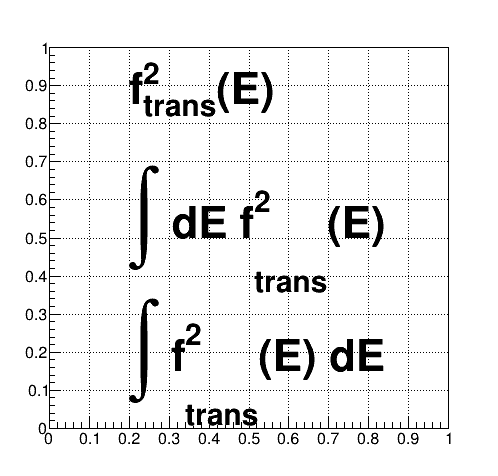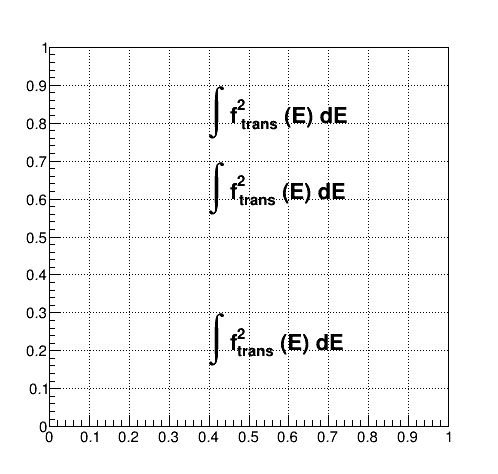# TLatex: subscript far below with integral

Hi…

The following code fragment

``````{
TCanvas TL("TL","TL", 500,500);
TL.SetGrid();
TL.DrawFrame(0,0,1,1);
TLatex latex;
latex.SetTextSize(0.1);
latex.DrawLatex(0.2, 0.85, "f^{2}_{trans}(E)");
latex.DrawLatex(0.2, 0.50, "#int dE f^{2}_{trans}(E)");
latex.DrawLatex(0.2, 0.15, "#int f^{2}_{trans}(E) dE");
TL.Print("intTlatex.png");
}
``````

yields this:TLatex subscripts go far below the baselines of their respective texts when used with `#int`; rather, it seems the subscripts were aligned to the baselines of integral characters…
How can I avoid this and make the positions of subscripts correct?

Kazuyoshi

ROOT Version: 6.23/01
Platform: linuxx8664gcc
Compiler: c++ (GCC) 10.1.1 20200507 (Red Hat 10.1.1-1)

Try adding a space and a negative space between the superscript and subscript:

`````` latex.DrawLatex(0.1, 0.4, "#int f^{2} #kern[-0.2]{ _{trans} (E) dE}");  // note the space before _{trans}
``````

or just:

`````` latex.DrawLatex(0.1, 0.4, "#int f^{2} #kern[-0.15]{_{trans} (E) dE}");
``````

https://root.cern/doc/v610/classTLatex.html#L11

A cleaner alternative: add empty limits on the integral:

`````` latex.DrawLatex(0.4, 0.8, "#int f^{2} #kern[-0.2]{ _{trans} (E) dE}");
latex.DrawLatex(0.4, 0.6, "#int f^{2} #kern[-0.15]{_{trans} (E) dE}");
latex.DrawLatex(0.4, 0.2, "#int^{}_{} f^{2}_{trans} (E) dE");
``````1 Like

Thanks!

Is this an intended feature? or a bug?

Maybe one of the developers can answer that, but they actually mention this (and the last solution I mentioned) in the same page I linked above, at the bottom of the “Subscripts and Superscripts” section (first section of the page).

1 Like

`#int` is implicitly waiting for limits. If you do not need them specify empty ones:

``````  latex.DrawLatex(0.2, 0.50, "#int^{}_{} f^{2}_{trans}(E) dE");
``````

This topic was automatically closed 14 days after the last reply. New replies are no longer allowed.